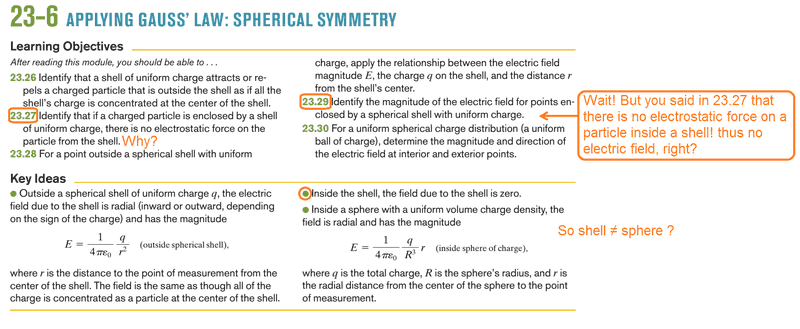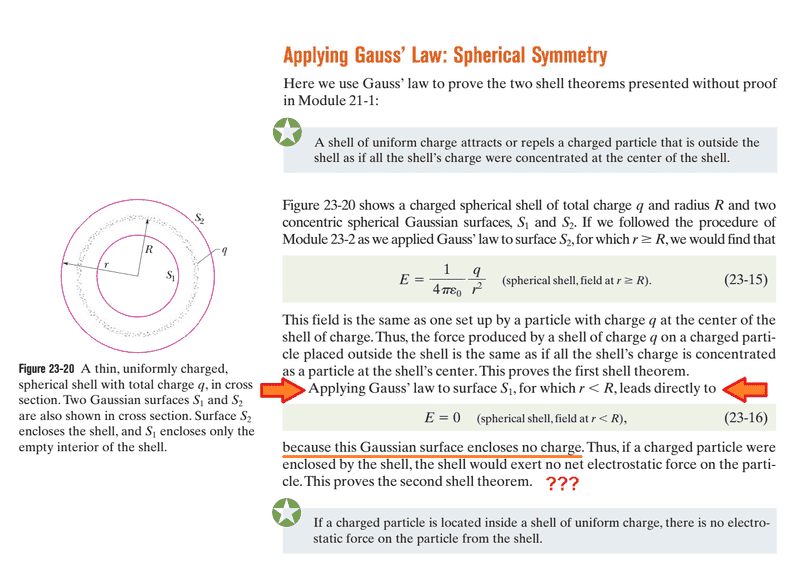Confusion over electric field inside a charged shell

I've got confused over a topic in my physics textbook (Fundamentals of Physics).
The textbook says if a charged particle is enclosed by a shell of uniform charge, there is no electrostatic force on the particle from the shell. I don't firmly get this, as the book uses a confusing reasoning for this. At first, the textbook makes a Gaussian surface called S1 inside the shell and it assumes there's no charge enclosed by S1, so according to Gauss' Law; there in no electric field inside the shell and S1 which I agree. However, just after that, the textbook concludes that there would be the same results if a charged particle was inside the shell. I don't know how this conclusion work.

I think a charged particle inside a charged spherical shell would produce an electric field in its nearby environment thus it would exert electrostatic forces on the shell (If I'm wrong, please prove it) therefore by Newton's third law, there will be the same force in magnitude exerted on the charged particle...jedishrfu
Mentor
This also applies to a shell of matter where there is no gravitational attraction inside the shell from the shell. If you were to integrate the forces from every point on the shell on the test charge they will sum to zero.

Here's a video description of it:

Is this an insulator shell or a conductor shell? The situations are different. For the conductive shell, the charge on the shell can re-distribute due to the extra charge inside so it won't be uniform any more.

This also applies to a shell of matter where there is no gravitational attraction inside the shell from the shell. If you were to integrate the forces from every point on the shell on the test charge they will sum to zero.

Here's a video description of it:

Thanks, but I watched the video but didn't get my answer.
I read those post but got more confused! I know that Gauss's law results that there would be no electric field inside the metal of a metallic shell, but why? what had exactly happened that resulted in no electric field? what canceled out?

Also, my textbook doesn't indicate if it's a conductor shell or an insulator like nasu asked."Applying Gauss’ law to surface S1, for which r is less than R, leads directly to E=0 because this Gaussian surface encloses no charge. Thus, if a charged particle were enclosed by the shell, the shell would exert no net electrostatic force on the particle."
I don't understand the sentence in orange. I think the particle exerts forces due to its electric field that induces charges on the shell (if it's conductor), so there should be a similar force on the particle from the shell according to Newton's third law. I also don't know how the book first proves that green text without the presence of any charged particles, but then concludes the same for a different situation with a charged particle inside?!

I read those post but got more confused! I know that Gauss's law results that there would be no electric field inside the metal of a metallic shell, but why? what had exactly happened that resulted in no electric field? what canceled out?

We know a prior that the E field is 0 inside a conductor in an electrostatic situation. If there were a field, charge would be moving. Since charge is not moving, there is no electric field. Sometimes Gauss's Law is used to find the field, knowing the charge enclosed. Sometimes, we know the field and use it to find the charge.

•